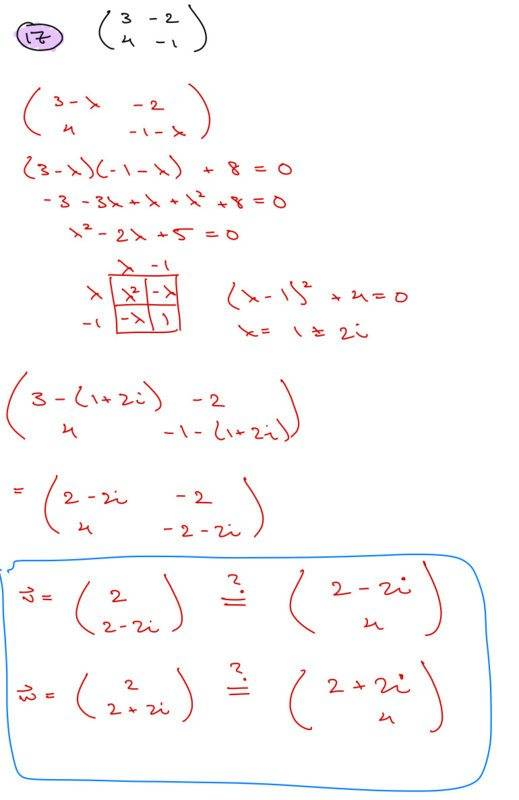# Find eigenvalues & eigenvectors

rugerts
Homework Statement:
Find eigenvalues & eigenvectors
Relevant Equations:
det(A-r*I) = 0Here's the problem along with the solution. The correct answer listed in the book for the eigenvectors are the expressions to the right (inside the blue box). To find the eigenvectors, I tried using a trick, which I don't remember where I saw, but said that one can quickly find eigenvectors (at least for simple 2x2 cases like these) by simply taking a row (of the matrix after having plugged in the eigenvalue) and shaping it such that the leftmost value is the first value for the eigenvector (with opposite sign) and the second eigenvector value is the same as the rightmost value. I heard that this is valid for either row choice... but when I do this, the answers appear to be different (hence the question mark over the inequality). So, my question is, are these eigenvectors shown in the blue box equivalent to one another? Does this trick really work? It seems to.
Thanks for your time.

## Answers and Replies

Homework Helper
Sort of. Those are the same two eigenvectors up to a constant, but in opposite order.

Multiply your ##\vec v## by the constant (1 + i). As you know, any multiple of an eigenvector is still an eigenvector of the same eigenvalue.
$$(1 + i) {2 \choose {2 - 2i}} = {{2 + 2i} \choose {2(1 - i)(1+i)}} = {{2+2i} \choose 4}$$
and similarly multiply ##\vec w## by the constant (1 - i).
$$(1 - i) {2 \choose {2 + 2i}} = {{2 - 2i} \choose {2(1 + i)(1-i)}} = {{2-2i} \choose 4}$$
Since the order is reversed, so are the eigenvalues with which each is associated.

•rugerts
Mentor
Also, you can verify that your eigenvalues and eigenvectors are correct or not by plugging them into the matrix equation and determining whether ##(A - \lambda I) \vec x = \vec 0##.

rugerts
Sort of. Those are the same two eigenvectors up to a constant, but in opposite order.

Multiply your ##\vec v## by the constant (1 + i). As you know, any multiple of an eigenvector is still an eigenvector of the same eigenvalue.
$$(1 + i) {2 \choose {2 - 2i}} = {{2 + 2i} \choose {2(1 - i)(1+i)}} = {{2+2i} \choose 4}$$
and similarly multiply ##\vec w## by the constant (1 - i).
$$(1 - i) {2 \choose {2 + 2i}} = {{2 - 2i} \choose {2(1 + i)(1-i)}} = {{2-2i} \choose 4}$$
Since the order is reversed, so are the eigenvalues with which each is associated.
Ahh, I see. Thank you